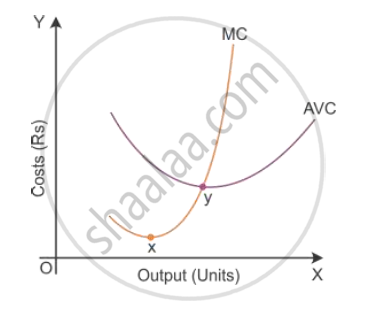Share

# State the Relation Between Marginal Cost and Average Variable Cost. - CBSE (Arts) Class 12 - Economics

#### Question

State the relation between marginal cost and average variable cost.

#### Solution

Average Variable Cost (AVC) and Marginal Cost (MC):
When AVC declines, MC also declines at a faster rate but below the AVC curve.
When AVC increases, MC also increases at a faster rate and remains above the AVC curve.
The MC curve cuts the AVC curve from its minimum point. Also, both AVC and MC are
obtained from TVC.

AVC = "TVC"/Q

MC = "ΔTC"/"ΔQ" = "ΔTVC"/"ΔQ"Is there an error in this question or solution?

#### APPEARS IN

Solution State the Relation Between Marginal Cost and Average Variable Cost. Concept: Cost - Average Variable Cost.
S